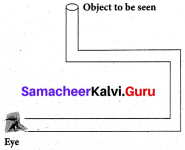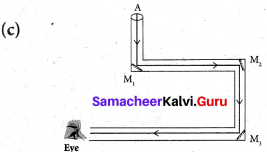Students can Download Science Chapter 1 Light Questions and Answers, Notes Pdf, Samacheer Kalvi 7th Science Book Solutions Guide Pdf helps you to revise the complete Tamilnadu State Board New Syllabus and score more marks in your examinations.

## Tamilnadu Samacheer Kalvi 7th Science Solutions Term 3 Chapter 1 Light

### Samacheer Kalvi 7th Science Light Textual Evaluation

I. Choose the correct option:

Light That Hits A Mirror Gets Question 1.
Light travels only in a ______ .It is because of this property that.
(c) straight line, reflection
(d) curved line and then straight line, shadows

Light That Hits A Mirror Gets Answer Question 2.
Light that hits a mirror gets ______
(a) Transmitted
(b) Reflected
(c) Absorbed
(d) Refracted
(b) Reflected

Samacheer Kalvi Guru 7th Science Question 3.
______ surface reflects the light well
(a) Water
(b) Compact disc
(c) Mirror
(d) Stone
(c) Mirror

Samacheer Kalvi 7th Science Guide Question 4.
Light is a form of ____
(a) matter
(b) energy
(c) medium
(d) partical
(b) energy

Light Samacheer Kalvi Question 5.
You can see your image in polished floors, but not in wooden table because ______
(a) regular reflection takes place in wooden table and irregular reflection in polished floor
(b) regular reflection takes place in polished floor and irregular reflection in wooden table
(c) regular reflection takes place in both polished floor and wooden table
(d) irregular reflection takes place in both polished floor and wooden table
(b) regular reflection takes place in polished floor and irregular reflection in wooden table

Samacheer Kalvi 7th Science Books Answers Question 6.
Choose the translucent substance from the following
(a) glass
(b) wood
(c) water
(d) Clouds
(d) clouds

Samacheer Kalvi 7th Science Question 7.
Reflection occurs , when the light
(a) about to reach a surface
(b) approaches a surface
(c) passes through a surface
(d) None Of these
(b) approaches a surface

7th Science Term 3 Question 8.
Which of the following is the best reflector
(a) plastic plate
(b) plane mirror
(c) wall
(d) paper
(b) plane mirror

Samacheer Kalvi 7th Science Answers Question 9.
Sivarajan placed a meter stick in the playground at 7.00 am in the morning. How will the shadow of the stick at noon look in comparison to the one in the morning
(a) There will be no shadow
(b) The shadow will be longer and on the opposite side as the sun
(c) The shadow will be shorter and on the same side as the sun
(d) The shadow will be shorter
(d) The shadow will be shorter

Samacheer Kalvi 7th Science Book Back Answers Question 10.
The image formed by a pinhole camera is inverted because,
(a) light travels in straight lines
(b) light rays become laterally inverted as they pass through a pinhole camera
(c) light rays pass through the pinhole
(d) light rays get reflected
(a) light travels in straight lines

7th Science Samacheer Kalvi Question 11.
Which of the following facts explain how shadows are formed?
(a) Light travels in straight lines
(b) Opaque bodies do not allow light to pass through them
(c) Reflection occurs at a smooth surfaces like mirrors
(d) Lateral inversion happens
(a) both A and B
(b) both A and D
(c) both B and C
(d) only A
(a) both A and B

II. Fill in the blanks:

1. A plane mirror produces a _________ image
2. A _________ reflection helps us to see the objects.
3. The light ray gets _________ when it falls on any polisher surface
4. Sunlight is a blend of _________ colours
5. The splitting of white light into seven colours is called _________
6. The moon _________ sun light.
7. The sunlight can be split into its constituent colours using _________
8. Reflection of light from rough surface is called _________ reflection

1. virtual and erect
2. regular
3. reflected
4. seven
5. dispersion
6. reflects
7. prism
8. irregular

III. Say TRUE or FALSE.

Samacheer Kalvi 7th Science Book Question 1.
The image of right hand in a plane mirror looks like a left hand.
True

7th Standard Term 3 Science Question 2.
Rainbow is formed by dispersion of which light by water drops.
True

Light Chapter Of Class 7 Pdf Question 3.
The image formed by the plane mirror is laterally inverted, hence the image seen through the periscope is also laterally inverted
False
Correct statement: The image formed by the plane mirror is laterally inverted, hence the image seen through the periscope is This is because in periscope, image is reflected by two mirrors.

Question 4.
We see planets because they reflect light from the sun
True

Question 5.
We see a book because it reflects the light that falls on its surface
True

Question 6.
The image formed in a pinhole camera is always inverted
True

Question 7.
The image formed in a pinhole camera is always the satne size as the object
False

Question 8.
The image formed in a plane mirror is upside down
False
Correct statement: The image formed in a plane mirror is erect.

Question 9.
A plane mirror is opaque Ans.
True

Question 10.
A shadow is formed on the same side of the object as the source of light.
False
Correct statement: A shadow is formed on the side of the object as the source of light.

Question 11.
We are able to see things around us with the help of regular reflection
True

Question 12.
After passing through a prism, white light splits into a band of seven colours
True

IV. Match the following

 1 Rectilinear propagation Primary source of light 2 Rectilinear propagation Non-luminous object 3 Plane Mirror Fire fly Periscope 4 The Moon Pinhole camera 5 Wide light source Spectrum of light 6 Regular reflection luminous object 7 The sun Penumbra 8 Band of seven colors Glossy surface

 1 Rectilinear propagation Pinhole camera 2 Rectilinear propagation Periscope 3 Plane Mirror Fire fly luminous object 4 The Moon Non-luminous object 5 Wide light source Penumbra 6 Regular reflection Glossy surface 7 The sun Primary source of light 8 Band of seven colors Spectrum of light

V. Answer the following questions in short:

Question 1.
With the help of a diagram, state the laws of reflection
Laws of reflection: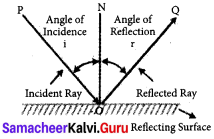1. The angle of incidence is always equal to the angle of reflection li =l*
2. The incident ray, the reflected ray and the normal at the point of incidence lie on the same plane.

Question 2.
Figure shows a pencil placed above a mirror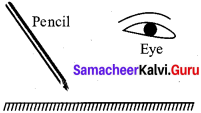a) Draw its image formed by the mirror
b) Show how light rays from the object are reflected at the mirror to form the image for the eye.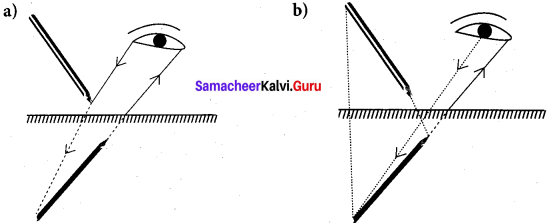Question 3.
A person is looking at the image of a tree in a mirror placed 3.5 m in front of him. Given that the tree is at 0.5 m behind his eyes. Find the distance between the image of the tree and his eyes. What are needed to see an object?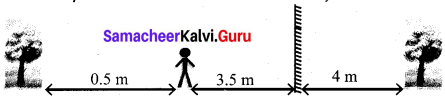Distance between the person and the mirror = 3.5 m
Distance between the person and tree (object) = 0.5 m
The image formed in the mirror = 4 m
The distance between the image of the tree and his eyes = 4 + 3.5 = 7.5

Question 4.
What are luminous objects?
All objects which emit light energy by themselves are called luminous objects. Ex.: Sun, electric bulb.

Question 5.
Is thr moon a luminous object?
No, the moon is non-luminous. The reason is that moon does not produce its own light. Instead, it reflects the light of the sun falling on it.

Question 6.
What are the three types of materials based on the absorption of light?

1. Transparent Material
2. Translucent Material
3. Opaque Material

Question 7.
What are the parts of shadow?

1. Umbra
2. Penumbra

Question 8.
What are the properties of shadow?

1. All objects do not form shadows. Only opaque objects form shadows.
2. Shadows will be formed in the opposite side of light source.
3. It cannot be determined the characteristics of an object by its shadow.
4. The shadow will be always darker, whatever may be the color of light rays.
5. Light source, opaque object are shadow all are in a straight line.
6. The size of shadow depends upon the distance between light source and object and the distance between object and the screen.

Question 9.
What is plane mirror?
A plane mirror is a mirror with a flat reflective surface. A plane mirror makes an image of objects in front of it.

Question 10.
What is prism?
A prism is an object made up of a transparent material, like glass or plastic that has at least two flat surfaces that form an acute angle (less than 90°).

Question 11.
What do you mean by visible light?
Visible light is a spectrum of a number of waves with different wavelength range from 400nm to 700nm (lnm = 10″9 meter) each wave has a definite wavelength represents a particular colour.

Question 12.
Write the items given here in the correct column (Stars, brick walls, plants, mirror, planets, electric light bulb, candle)

 Sources of Light Objects that reflect light

 Sources of Light Objects that reflect light Stars Brick walls Electric light bulb Plants Candle Mirror Planets

Question 13.
A boy of height lm 45 cm is standing in front of a long mirror at a distance of 2 m. From this information, fill up the following sentences:
a) The distance between the boy and his image is ______
b) The height of the image is ______
c) When the boy moves 1 m forward, the distance between her and her image is ______
(a) The distance between the boy and his image is 4m
(b) The height of the image is same.
(c) When the boy moves 1 m forward, the distance between her and her image is 2m.

Question 14.
Draw a diagram of a pinhole camera showing the rays of light passing between the Object and its image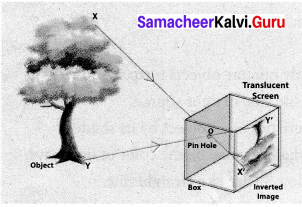Question 15.
Why is the writing on the front of an ambulance back to front as shown in the picture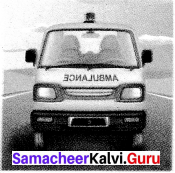1. This is due to lateral inversion.
2. The phenomenon due to which the left side of an object appears to be right side of the object in its image in a reflecting medium (mirror).
3. So that drivers see the word the right way around in their rear-view mirror.

Question 16.
Explain with examples, why some capital letters look the same in a mirror but others are reversed.
Any letter that has a bilateral symmetry will have its mirror image the same as that of the object.
Example: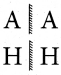Question 17.
Two plane mirrors M1 and M2 are placed perpendicular with each other, as shown in figure. The ray AB makes an angle 39° with the plane mirror M , then

1. The reflected rays are _________, ____________
2. The incident rays are _________, ____________
3. What is the angle of incident corresponding to the ray BC?
4. What is the angle of reflection corresponding to the ray CD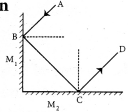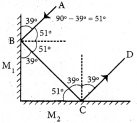1. BC, CD
2. AB, BC
3. $$\lfloor i$$ = 39°
4. $$\lfloor r$$ = 39°

Question 18.
Raj an was playing with the mirror images of a clock. He looked at the clock in his roomIt was showing 1:40. Draw the position of the hands on the real clock and on its mirror reflection .Write below the picture what time each picture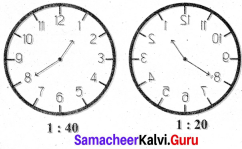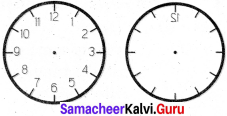Question 19.
What is reflection of light?
The bouncing back of light from a reflecting surface of an object is called as reflection of light

Question 20.
If a ray of light is falling on a plane mirror at an angle of 50° is formed, what will be the angle of reflection?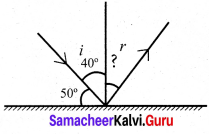Angle of incidence $$\lfloor i$$ = 90° – 50°
$$\lfloor i$$ = 40°
According to laws of reflection,
$$\lfloor i$$ = $$\lfloor k$$
Angle of incidence = Angle of reflection
$$\lfloor i$$ = 40°
∴ $$\lfloor r$$ – 40°
Angle of reflection $$\lfloor r$$ = 40°

Question 21.
What do you mean by lateral inversion?
The phenomenon due to which left hand side of object appears as right hand side and vice versa is called lateral inversion.

Question 22.
How do you obtain a spectrum of light?
When white light is made to fall on the surface of a prism, it disperses and we obtain a spectrum of light.

Question 23.
Why do we see white color in Newton’s disc, when we rotate it very fast?
When the disc turned quickly, the retina receives the sensation of the spectrum simultaneously and disc appears white.

Question 24
What is a shadow? What things are necessary for the formation of a shadow?

1. Shadows are formed because light travels in straight lines.
2. Shadow is always against, opposite side of light source.
3. Shadow is formed by opaque objects that stop high from propagating.
4. Things necessary for the formation of shadow: source of light,opaque object

VI. Answer the following questions in detail:

Question 1.
What are regular and irregular reflection? Explain with the help of diagrams
(i) Regular reflection When a parallel beam of light on striking some smooth and polished surface is reflected as a parallel beam of light, such a reflection is called regular reflection.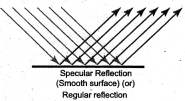(ii) Irregular reflection : When a parallel beam of light, on striking some rough surface, is reflected in different directions, then such a reflection is called irregular or diffused reflection.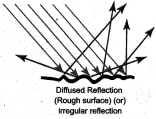Question 2.
What are the difference between luminous and non-luminous objects? Give two examples of each.

 Luminous objects Non-luminous objects 1. All objects which emit light energy by themselves are called luminous objects. All objects which do not emit light energy of their own, but reflect the light energy falling on them and hence, become visible are called non- luminous object. 2. Ex.: Sun, stars, torch light, burning candle etc. Ex.: Metals, trees, houses, stones, moon etc.

Question 3.
Write about two everyday situations that tell you that light travels in a straight line.

1. Formation of shadow: Shadows are formed when some light rays continue its travel in straight lines while other rays are stopped by an object.
2. When there is a small hole in a room, light travels only in a straight line.

Question 4.
Differentiate between a reflection and a shadow

 Reflection Shadow When light falls on a surface, the direction of ray is changed. This change in direction is known as reflection of light. Shadow is an image formed by obstruction of light. Eg.: You see your reflection in a pool of water or mirror. Eg.: If you place your hand in front of a candle, you will see the image of the hand on the wall. This image will show only the outline of the object placed in front of the light source.

Question 5.
What are the characteristics of an image formed in a plane mirror?

1. Image formed in a plane mirror is upright.
2. Image formed in a plane mirror is virtual The image is of the same size as the object
3. The distance of the image from the plane mirror is equal to the distance of the object from the mirror.
4. Image is laterally inverted.

Question 6.
Describe the picture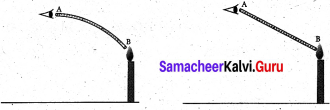1. When the tube is bent, we cannot see the source of light
2. When the tube is held straight we can see the flame. The two pictures verify that light travels in straight lines. This is known as rectilinear propagation of light.

Question 7.
Define the following terms
a. Incident ray
b. Reflected ray
c. Normal
d. Angle of incidence
(a) Incident tavi The ray of light that falls on the surface of the reflection materials.
(b) Reflected ray: The ray of light that comes from the point when the incident ray falls on the reflection material.
(c) Normal: The perpendicular line drawn from, the point of incidence to the plane of reflecting surface is called normal.
(d) Angl e of incidence The angle formed between the incident ray PO and the normal ‘ON’ is angle of incidence.

Question 8.
Compare the images formed by plane mirror with that by pinhole camera
Difference between the images formed in Pinhole camera and Plane mirror:

 Image formed by Pin hole camera Images formed in Plane mirror The image is real. The image is virtual The image may not be equal to the size of the object The image is equal to the size of the object The image is inverted The image is erect

### Samacheer Kalvi 7th Science Light Intext Activities

Activity – 4

Let’s categorize transparent material, translucent material and opaque material among f the given materials
(Clear plastic ruler, cellophane paper, some water in a glass jar, tissue paper, drinking glass, beaker, tap water, kerosene, coconut oil, note paper, card board, milk, diluted milk, aluminum foil, thick colored plastic lid, rough glass piece, measuring glass with water, wooden piece) Place all the materials given above in the dark room. Focus a torch light on one side of each material. Inspect the light coming out at the other side of each material and then classify the materials in the table.

 S.No Transparent Material Translucent Material Opaque Material 1. Clear plastic ruler Kerosene Card board 2. Drinking glass Milk Wooden piece 3. Beaker diluted milk aluminium foil 4. Tap water rough glass piece thick coloured plastic lid. 5. Measuring glass with water tissue paper 6. Cell phone paper Note paper

Activity – 6

 Surfaces Property of Image (Clearer / blurred) Glass Blurred Metal Sheet Blurred Aluminum foil Clearer Whitepaper Clearer

Activity – 7

There are eight letters in the word EINSTEIN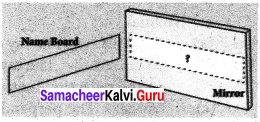1. Write the word in front of a plane mirror shown in diagram
2. Write down how these letters appear in the mirror
3. How many of these letters appear to be different, when the word is reflected?
4. Write down the letters that appear to be the same.

(i)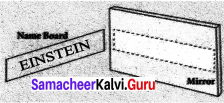(ii)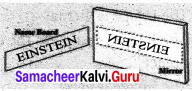(iii) 3 letters (E,N,S)
(iv) I,T

Activity – 10
You need Gelatin papers of Red, Blue and Yellow. Fold each gelatin paper three times and look different colour objects listed below through each folded paper. Observe what colour each object has. Write your observations in the table.

 Object Original colour of object Colour through Red gelatin paper Colour through Blue gelatin paper Colour through Yellow gelatin paper Blue sky Blue Orange flower Orange Yellow banana Yellow leaves Green Brown trouser Brown White shirt White Red Blue Yellow Black board Black

### Samacheer Kalvi 7th Science Light Additional Questions

Question 1.
Which of the following is a natural source of light?
(a) Electric lamp
(b) Electric filament
(c) Torch light
(d) Sunlight
(d) Sunlight

Question 2.
Light shows ________
(a) curvilinear propagation
(b) random propagation
(c) rectilinear propagation
(d) none of these
(c) rectilinear propagation

Question 3.
Rectilinear propagation is ________
(a) mode of travelling in straight lines
(b) mode of travelling in curved lines
(c) ability to bend around obstacles
(d) displaying the phenomenon of diffraction
(a) made of travelling in straight lines

Question 4.
Plane mirrors are arranged parallel to each other to get ________
(a) a single image
(b) two images
(c) a large number of reflected image
(d) no image
(d) two images

Question 5.
Which of the following is not luminous object?
(a) Sun
(b) Moon
(c) Candle
(d) Bulb
(b) Moon

Question 6.
White light is composed of ________
(a) seven colours
(b) five colours
(c) three colours
(d) only one colours
(a) seven colours

Question 7.
The image which can be obtained on screen is called ________
(a) erect
(b) real
(c) virtual
(d) inverted
(b) real

Question 8.
If we mix lights of the colours of the rainbow we will get .
(a) pink light
(b) brown light
(c) colourless light
(d) black light
(c) colourless light

Question 9.
Which one of the following is involved for the formation of rainbow in the sky?
(a) Expansion of light
(b) Expansion of heat by the Sun
(c) Distance between the Earth and the Moon
(d) Dispersion of light
(d) Dispersion of light

Question 10.
A virtual image is
(a) always real
(b) always uncapturable on a screen
(c) always inverted
(d) always caught on a screen
(b) always uncapturable on a screen

II. Fill in the Blanks.

1. Objects which are able to emit light are known as ______
2. Passing electricity through certain gases at a very low pressure can produce ______
3. ______ is a simple device which helps us to understand about the rectilinear propagation of light.
4. The light falling on the mirror is called ______ as and the light reflected is called
5. The angle formed between the reflected ray and the normal is ______
6. Light travels ______ km per second in air or vacuum.
7. Materials that allow light to pass through completely are known as ______ material.
8. Materials that allow light to pass through partially are called ______ materials.
9. Materials that are not able to allow light to pass through it are called ______ materials.
10. ______ is always against, opposite side of light source.
11. The ______ is the darkest part of a shadow.
13. Only ______ objects forms shadows.
14. ______ eclipse occurs, when the moon arrives between the sun and the Earth.
15. ______ cables are able to carry more signals than traditional copper cable telephone lines.
16. The images that are obtained on a screen are called ______ and that which cannot be obtained on a screen is ______
17. When all colours of visible light strikes the retina of our eye at the same time, our brain perceives ______
18. ______ colour is scattered the least by air molecules.
19. ______ is the method of creating colour by mixing various proportion of two or three distinct colours of light.
20. ______ Magenta, Cyan and yellow are called colours.

1. light sources
2. Light
3. Pin-hole camera
4. incident ray, reflected ray
5. angle of reflection
6. 3 lakh
7. transparent
8. translucent
9. opaque
11. umbra
12. penumbra
13. opaque
14. Solar
15. Fibre optic
16. real image, virtual image
17. white
18. Red
19. Synthesis
20. secondary

III. True or False – If false give the correct statement.

Question 1.
Angle of incidence is greater than the angle of reflection.
False
Correct statement: Angle of incidence is o the angle of reflection.

Question 2.
The image formed by a plane mirror is real.
False.
Correct statement: The image formed by a plane mirror is virt ual and erect.

Question 3.
Diffused reflection gives a sharp image.
False.
Correct statement: reflection gives a sharp image.

Question 4.
The image formed by a plane mirror is always that far behind the mirror as far the object lies in front of it.
True.
You can see a rainbow only when your back is towards the sun.
True.

Question 5.
You can see a rainbow only when your back is towards the sun.
True.

Question 6.
The image of symmetrical letters like A, H, I, M, R, K, T, U are not affected by lateral inversion.
Correct statement: The image of symmetrical letters like A, H, I, M, T, U are not affected by lateral inversion.

Question 7.
Plane mirrors are used as looking glass, to reflect light on objects and in periscopes.
True

Question 8.
We can not get white light when we mix seven colours.
False
Correct statement: We can get white light when we mix seven colours.

Question 9.
Materials that allow light to pass through completely are known as transparent material
True

Question 10.
Solar and lunar eclipses are occuring due to the property of light known as the rectilinear propagation of light.
True

IV. Match the following :

Question 1.

 1. Rubber (a) Erect 2. Greased paper (b) Inverted 3, Real image (c) Opaque medium 4. Virtual image (d) Translucent medium

1. c
2. d
3. b
4. a

Question 2.

 1 Real image (a) Diffuse reflection 2 Virtual image (b) An optical device which produces reflection 3 Mirror (c) Cannot be taken on screen 4 A type of light scattering (d) Can be taken on screen

1. d
2. c
3. b
4. a

V. Assertion and Reason.

Mark the correct choice as
(a) Both A and R are true and R is the correct explanation of assertion.
(b) Both A and R are true but R is not the correct explanation of assertion.
(c) A is true but R is false.
(d) A is false but R is true.

Question 1.
Assertion (A) : A plane mirror produces virtual, erect image for any position of the object.
Reason (R) : Lateral inversion is an important property of image formed by a plane mirror.
(b) Both A and R are true but R is not the correct explanation of assertion.
Correct reason: It is because of the property of image formed in a plane mirror.

Question 2.
Assertion (A) : When an object is placed between two plane mirrors; then all the images
found are of unequal intensity.
Reason (R) : In case of plane parallel mirrors, only two images are possible.
(c) A is true but R is false
Correct reason: In case of plane parallel mirrors, infinite images are formed.

Question 3.
Assertion (A) : Virtual object can’t be seen by human eye.
Reason (R) : Virtual image is formed by converging rays.
(c) A is true but R is false
Correct reason: Virtual image is formed by diverging rays.

Question 4.
Assertion (A) : A ray incident along normal to the mirror retraces its path.
Reason (R) : In reflection, angle of incidence is always equal to angle of reflection.
(a) Both A and R are true and R is the correct explanation of assertion

Question 1.
What is the composition of sunlight?
Sunlight is a mixture of seven colours.

Question 2.
How does light travel?
Light travels along a straight line.

Question 3.
What kind of surface reflects light?
Smooth and shiny surface reflects light.

Question 4.
Name the triangular piece of glass that splits white light into different colours.
Prism.

Question 5.
What is reflection of light?
When light falls on smooth surface like mirror then it changes its direction, it is called reflection.

Question 6.
State the size of the image formed by a plane mirror?
The size of image formed by a plane mirror is same as that of the object infront of the mirror.

Question 7.
What is Newton’s disc?
Newton Disc is a card board disc with seven equal sectors colored red, yellow, orange, green, blue, indigo and violet. When the disc turned quickly, the retina receives the sensation of the spectrum simultaneously and disc appears white.

Question 8.
What is an eclipse?
An eclipse is an incident, when any astronomical object is partially or fully obscured due to the placement of another astronomical object in the presence of light.

Question 9.
What is light?
Light is type of energy, that helps us to see all the things around us.

Question 1.
What is natural sources of light? Give example.
Sources which emit light naturally are known as natural sources of light. The Sun is the primary and the major source of natural light.

Question 2.
Mention the properties of light.
Light has some fundamental properties as mentioned below.

• Rectilinear propagation of light
• Reflection
• Speed
• Interaction of light with matter.
1. Types of material according to permeability.
3. Plane mirror and images.
4. Spectrum.

Question 3.
State laws of reflection.
Laws of reflection:

1. The angle of incidence is always equal to the angle of reflection. $$\lfloor i$$ = $$\lfloor r$$
2.  The incident ray, the reflected ray and the normal at the point of incidence lie on the same plane.

Question 4.
Define transparent materials. Give example.

1. Materials that allow light to pass through completely are known as transparent material.
2. Example: Eye glasses, clear drinking glass, clear water, face glasses used in buses.

Question 5.
Define opaque material. Give example.

1. Materials that are not able to allow light to pass through, are called opaque material.
2. Example: Wall, thick card board, stone, etc.

Question 6.
What is lunar eclipse?

1. Lunar eclipse occurs, when the Earth (E) comes between the Sun (S) and the Moon (M).
2. The Earth prevents light coming from the Sun and makes shadow on the Moon. This is lunar eclipse.

Question 7.
Why danger lights in vehicles are red in colour?

1. Red color is scattered the least by air molecules.
2. Red color has the highest wavelength of all the other colors. So red color is able to travel the longest distance through air, fog.

Question 8.
What is meant by synthesis of colour?

1. Synthesis of colour is the method of creating colour by mixing various proportion of two (or) three distinct colours of light. .
2. These distinct colours are Red, Green and Blue called as primary colours.

Question 9.
When lighting a bulbs in a dark room, light spreads the whole room quickly. Give reason.

1. When lighting a bulb in a dark room, light spreads the whole room quickly.
2. This is because the light travels very fast.
3. Light travels three lakh kilometers per second in air or vacuum.
4. In theory, nothing can travel faster than light.

Question 1.
Write the difference between real image and virtual image.

 Real image Virtual image 1. It can be taken on a screen. It cannot be taken on a screen. 2. The rays of light, after reflection The rays of light, after reflection appear to 3. It is always inverted It is always erect but laterally inverted

Question 2.
Explain the importance of optical fiber.

1. Optical fiber is a device that works on the principle of total internal reflection by which light signals (huge data) can be transmitted from one place to another place with a negligible loss of energy in a very short time.
2. It consists of a cable having one or more thin flexible fibers with a glass core through which light signals can be sent.
3. Optical fiber can be twisted and bent easily.
4. When a light a ray of light is incident at one end of the core of optical fiber, it suffers total internal reflection at the many places inside the fiber and emerges at the other end with negligible loss of energy.
5. The data or information in the form of pulses of light, can be sent through bundles of optical fibers.
6. Optical fibers have become very important in high-speed communications, such as cable TV and high-speed broadband services.Fiber optic cables are able to carry more signals than traditional copper cable telephone lines.

Question 3.
With help of a diagram, write a note on : a. Solar eclipse, b. Lunar eclipse.
(a) Solar eclipse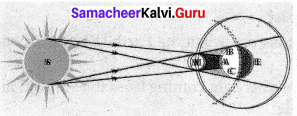Solar eclipse occurs, when the Moon arrives between the Sun (S) and the Earth(E). The shadow of the Moon appears on the Earth at A as shown in picture. Hence, those who are at the region A are unable to see the Sun instantly. This is solar eclipse. But, those who are at the region B and C are able to see the sun partially.
(b) Lunar eclipse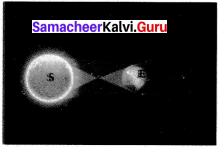Lunar eclipse: Lunar eclipse occurs, when the Earth (E) comes between the Sun (S) and the Moon (M). The Earth prevents light coming from the Sun and makes shadow on the Moon. This is lunar eclipse

IX. Problems for practice:

Question 1.
Calculate the number of images formed by plane mirrors kept at an angle of 10° to each others.
Solution:
Number of image n = $$\frac { 360° }{ θ}$$ – 1 = $$\frac { 360° }{ 10°}$$ – 1
= 36 – 1
= 35 images

Question 2.
A ray of light fall on a plane surface at an angle of incidence 9° and reflection occurs. Calculate the angle of reflection in degree.
Solution:
By first law of reflection, angle of incidence is always equal to angle of reflection.
$$\lfloor i$$ = $$\lfloor r$$ . Here, angle of incidence is 9°. So angle of reflection must be equal to 9°.

Question 3.
A plane mirror forms a virtual image. The distance between Maria and her image in a plane mirror is 10 m. How much distance should she move in order to get the distance of 5 m between herself and her image?
Solution: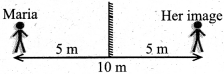The distance between Maria and her image in a plane mirror = 10 m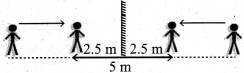So, she shouuld move a distance of 2.5 m toward the plane mirror

X. Higher order thinking skill (HOTS) :

Question 1.
Why are we able to see the tap water in a glass even though it is colourless?
Top water reflects the light into our eyes. As long as an object reflects light into our eyes, we can see it.

Question 2.
Imagine that parallel rays are incident on an irregular surface. Are the rays reflected from this surface parallel to each other?